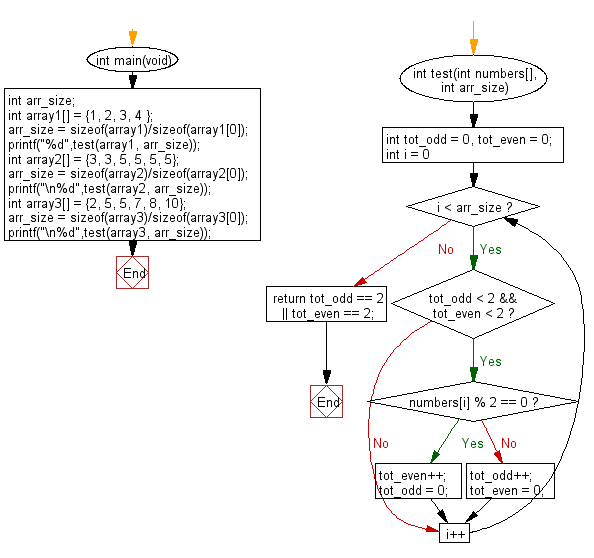﻿ C : 2 even or 2 odd values next to each other in an array# C Exercises: Check a given array of integers and return true if the given array contains either 2 even or 2 odd values all next to each other

## C-programming basic algorithm: Exercise-63 with Solution

Write a C program to check a given array of integers and return true if the given array contains either 2 even or 2 odd values all next to each other.

C Code:

``````#include <stdio.h>
#include <stdlib.h>

int main(void){
int arr_size;
int array1[] = {1, 2, 3, 4 };
arr_size = sizeof(array1)/sizeof(array1);
printf("%d",test(array1, arr_size));
int array2[] = {3, 3, 5, 5, 5, 5};
arr_size = sizeof(array2)/sizeof(array2);
printf("\n%d",test(array2, arr_size));
int array3[] = {2, 5, 5, 7, 8, 10};
arr_size = sizeof(array3)/sizeof(array3);
printf("\n%d",test(array3, arr_size));
}

int test(int numbers[], int arr_size)
{
int tot_odd = 0, tot_even = 0;

for (int i = 0; i < arr_size; i++)
{
if (tot_odd < 2 && tot_even < 2)
{
if (numbers[i] % 2 == 0)
{
tot_even++;
tot_odd = 0;
}
else
{
tot_odd++;
tot_even = 0;
}
}
}
}
``````

Sample Output:

```0
1
1
```

Pictorial Presentation:Flowchart:C Programming Code Editor:

What is the difficulty level of this exercise?

Test your Programming skills with w3resource's quiz.

﻿

## C Programming: Tips of the Day

Why doesn't a+++++b work?

printf("%d",a+++++b); is interpreted as (a++)++ + b according to the Maximal Munch Rule!.

++ (postfix) doesn't evaluate to an lvalue but it requires its operand to be an lvalue.

! 6.4/4 says the next preprocessing token is the longest sequence of characters that could constitute a preprocessing token"

Ref : https://bit.ly/3fdldUT